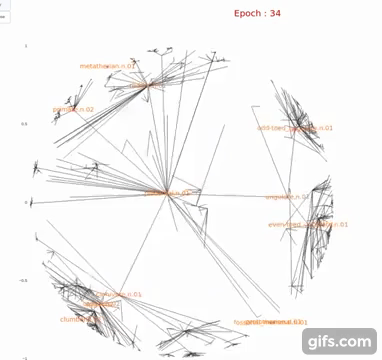# Curious Case of Hyperbolic Embeddings (Part - I )LEFT : Euclidean Embedding || RIGHT : Hyperbolic Embedding (Poincare Embedding)
1. What are the possible metric spaces where embedding is possible ?
2. What are the constraints of Euclidean Spaces ?
3. Why should you even consider using Hyperbolic Embeddings ?
4. Real Scenarios where Hyperbolic Embeddings prove useful in comparison with Euclidean embedding .
5. What are it’s limitations ?
6. What would be the next step for Hyperbolic Embeddings ?
7. Multi-dimensional Implementation of Hyperbolic Embeddings using Gensim and Plotly
1. Riemannian Manifolds
2. Graph Metrics
1. Genomics (Human Protein Interactions)
2. Bioinformatics (Cancer Networks)
3. Recommender Systems
4. Network Science (Internet Topology)
5. Preserving Privacy

One of the few notable impacts of Impacts f Hyperbolic Embeddings are :

• Drastic reduction in number of parameters and embedding dimensionality
• Better disentanglement in embedding space : Improved clustering, Classification, interpret ability
• Better model understanding and interpretation
• Shape :
1. Basic operations such as adding 2 elements or re-scaling 1 by a real number are not available in Hyperbolic Embeddings

# IMPLEMENTATION

I have created a Knowledge Graph of Hyperbolic Word Embedding using Gensim Library.

`# extract subjectsource = [i for i in entity_pairs]# extract objecttarget = [i for i in entity_pairs]kg_df = pd.DataFrame({'source':source, 'target':target, 'edge':relations})# creating a directed-graph from a dataframe using Networkx libraryG=nx.from_pandas_edgelist(kg_df, "source", "target",                           edge_attr=True,                          create_using = nx.MultiDiGraph())plt.figure(figsize=(12,12))pos = nx.spring_layout(G)nx.draw(G, with_labels=True, node_color='skyblue', edge_cmap=plt.cm.Blues, pos = pos)plt.show()`
`G=nx.from_pandas_edgelist(kg_df[kg_df['edge']=="thought"], "source", "target",                           edge_attr=True, create_using=nx.MultiDiGraph())plt.figure(figsize=(12,12))pos = nx.spring_layout(G, k = 0.5) # k regulates the distance between nodesnx.draw(G, with_labels=True, node_color='skyblue', node_size=1500, edge_cmap=plt.cm.Blues, pos = pos)plt.show()`
`from gensim.models.poincare import PoincareModelnew_model = PoincareModel(train_data = entity_pairs,                          size = 2,                          burn_in = 0,                          workers = 1,                          negative = 10)from gensim.viz.poincare import poincare_2d_visualization, poincare_distance_heatmapfig = poincare_2d_visualization(    new_model,    entity_pairs,    "Poincare Hierarchy",    show_node_labels=entity_pairs)`
`x = new_model_without_2d.kv.most_similar('Alice', topn = 10)`

# REFERENCES :

1. Hyperbolic Deep Learning (http://hyperbolicdeeplearning.com/)
2. Dissertation of Octavian Ganea (https://ei.is.tuebingen.mpg.de/publications/tifgecgan19)
3. Poincare(Hyperbolic) Implementation of Gensim Library (https://radimrehurek.com/gensim/models/poincare.html)
4. My Implementation using Poincare models of Gensim and Plotly (https://github.com/123nishant/Hyperbolic-Embedding/blob/main/Hyperbolic%20Embeddings%20in%203D.ipynb)

Written by